# PSEB 7th Class Maths Solutions Chapter 2 Fractions and Decimals Ex 2.2

Punjab State Board PSEB 7th Class Maths Book Solutions Chapter 2 Fractions and Decimals Ex 2.2 Textbook Exercise Questions and Answers.

## PSEB Solutions for Class 7 Maths Chapter 2 Fractions and Decimals Ex 2.2

1. Match the following :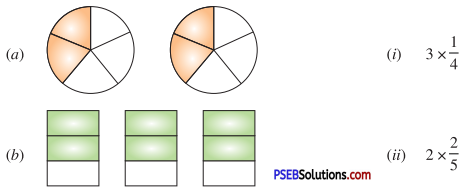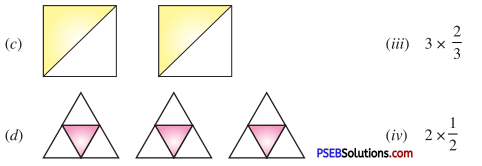(a) (ii),
(b) (iii),
(c) (iv),
(d) (i).2. Multiply whole number to a fraction and reduce it to the lowest form. Also convert into a mixed fraction :

Question (i).
4 × $$\frac {1}{3}$$
4 × $$\frac {1}{3}$$ = $$\frac{4 \times 1}{3}$$
= $$\frac {4}{3}$$
= 1$$\frac {1}{3}$$

Question (ii).
11 × $$\frac {4}{7}$$
11 × $$\frac {4}{7}$$ = $$\frac{11 \times 4}{7}$$
= $$\frac {44}{7}$$
= 6$$\frac {2}{7}$$

Question (iii).
$$\frac {3}{4}$$ × 6
$$\frac {3}{4}$$ × 6 = $$\frac{3 \times 6}{4}$$
= $$\frac {9}{2}$$
= 4$$\frac {1}{2}$$

Question (iv).
$$\frac {9}{7}$$ × 5
$$\frac {9}{7}$$ × 5 = $$\frac {45}{7}$$
= 6$$\frac {3}{7}$$

Question (v).
2$$\frac {5}{6}$$ × 4
2$$\frac {5}{6}$$ × 4 = $$\frac{17}{6} \times 4$$
= $$\frac{17 \times 4}{6}$$
= $$\frac{17 \times 2}{3}$$
= $$\frac {34}{3}$$
= 11$$\frac {1}{3}$$

Question (vi).
10$$\frac {5}{6}$$ × 5
10$$\frac {5}{6}$$ × 5
= $$\frac {65}{6}$$ × 5
= $$\frac{65 \times 5}{6}$$
= $$\frac {325}{6}$$
= 54$$\frac {1}{6}$$Question (vii).
5 × 6$$\frac {3}{4}$$
5 × 6$$\frac {3}{4}$$ = 5 × $$\frac {27}{4}$$
= $$\frac{5 \times 27}{4}$$
= $$\frac {135}{4}$$
= 33$$\frac {3}{4}$$

Question (viii).
3 $$\frac {2}{5}$$ × 8
3 $$\frac {2}{5}$$ × 8 = $$\frac {17}{5}$$ × 8
= $$\frac{17 \times 8}{5}$$
= $$\frac {136}{5}$$
= 27$$\frac {1}{5}$$

3. Solve :

Question (i).
$$\frac {1}{2}$$ of 46
$$\frac {1}{2}$$ of 46
= $$\frac {1}{2}$$ × 46
= 23

Question (ii).
$$\frac {2}{3}$$ of 27
$$\frac {2}{3}$$ of 27
= $$\frac {2}{3}$$ × 27
= 18

Question (iii).
$$\frac {1}{3}$$ of 36
$$\frac {1}{3}$$ of 36
= $$\frac {1}{3}$$ × 36
= 12

Question (iv).
$$\frac {3}{4}$$ of 16
$$\frac {3}{4}$$ of 16
= $$\frac {3}{4}$$ × 16
= 12

Question (v).
$$\frac {5}{7}$$ of 35
$$\frac {5}{7}$$ of 35
= $$\frac {5}{7}$$ × 35
= 25(i) $$\frac {1}{3}$$ of the rectangles in box (a)
(ii) $$\frac {2}{3}$$ of the circles in box (b)
(iii) $$\frac {1}{2}$$ of the triangles in box (c)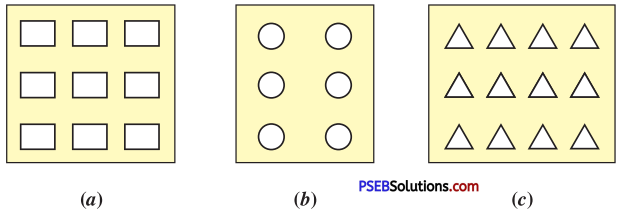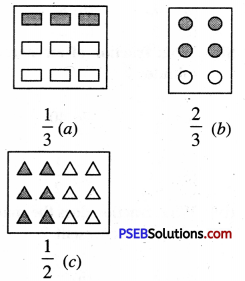5. Rahul earns ₹ 44,000 per month. He spends $$\frac {3}{4}$$ th of his income every month and saves the rest of his earning. Find his monthly savings ?
Rahul earns per month = ₹ 44000
He spends = $$\frac {3}{4}$$th of ₹ 44000
= ₹ $$\frac {3}{4}$$ × 44000
= ₹ 33000
His savings = ₹ 44,000 – ₹ 33000
= ₹ 11000

6. The cost of a book is ₹ 117$$\frac {1}{2}$$. Find the cost of 8 books ?
Cost of one book = ₹ 117 $$\frac {1}{2}$$
= ₹ $$\frac {235}{2}$$
Cost of 8 books = 8 × ₹ $$\frac {235}{2}$$
= ₹ 4 × 235
= ₹ 9407. Multiple choice questions :

Question (i).
$$\frac {1}{2}$$ × 8 is equal to
(a) 8
(6) 2
(c) 4
(d) 1
(c) 4

Question (ii).
$$\frac {3}{2}$$ of 16 is :
(a) 48
(b) 8
(c) 3
(d) 24
(d) 24

Question (iii).
What fraction of an hour is 40 minutes ?
(a) $$\frac {2}{3}$$
(b) 40
(c) $$\frac {1}{4}$$
(d) $$\frac {1}{2}$$
(a) $$\frac {2}{3}$$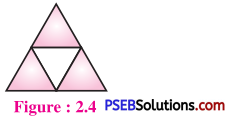(a) $$\frac {1}{3}$$
(b) $$\frac {2}{3}$$
(c) $$\frac {3}{4}$$
(d) $$\frac {1}{2}$$
(c) $$\frac {3}{4}$$